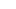# Text Functions - Advance Excel

October 10, 2014

There are hundreds of formulas in Excel. In this post I would like to cover some of the Text Functions in Excel.

Assumption: A1 has a word Upskill Technologies

Len: Len returns the number of characters in a string.

Ex: =Len(A1)  would return 20

Left: Left returns n number of characters from a string starting from left.

Ex: Left(A1,4) would return Upsk

Right: Right function returns 'n' number of characters from right.

Ex: Right(A1,4) would return gies

Mid: Mid function returns 'n' number of characters from a position specified.

Ex: Mid(A1,3,5) would return the characers skill

Find: Find functions returns the position of a character in a string.

Ex: Find("k",A1,1) would return 4

Ex: Find(" ",A1,1) would return 8

Now Combining all the above functions if you want to write a formula to get the 1st name that is to split cell A1 using space as delimter then we use something like below.

First Name: =LEFT(A1,FIND(" ",A1,1)-1) would return Upskill

The above formula find the position of space using find function which would return a value 8. And then Left formula gets the 7 characters(8 minus 1) from the left of cell A1.

Last Name: =RIGHT(A1,LEN(A1)-FIND(" ",A1,1)) will reutn Technologies

The above formula finds the characters from right after the position of space. Position of space is found using length of A1 i.e. 20 and position of space i.e.8 so remaining 12 characters from right.

Upper: Upper formula converts the text to upper case

Ex: Upper(A1) would return UPSKILL TECHNOLOGIES

Lower: Lower formula converts the text to lower case

Ex: Lower(A1) would return upskill technologies

Substitute: This substitutes replaces specific text in a string

Ex: SUBSTITUTE(A1," ","_",1) would return Upskill_Technologies

We learned in our classes the other practical uses of text functions and how to use them along with If conditions, vlookups,sumifs....

###### Featured PostsExcel Demo Video

November 14, 2014

1/2